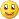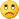# Martingale EA - page 81[Deleted]

BBop,

Could you start with posting your setfile first and I will try and help. I am not the creator of this EA but wolfe is not here yet...so I will try to help if that is ok with you...

Save your settings in the input diologue area...then go into explorer and copy and paste the original text file in experts/presets right here...I will highlight any changes that I can find in blue

ES

P.S. shit..your a coder...sorry to talk to you like you did not know....my mistake...75

As i said, i used initial settings...

Start_Lot_Size=0.10000000

Start_Lot_Size,F=0

Start_Lot_Size,1=0.10000000

Start_Lot_Size,2=0.00000000

Start_Lot_Size,3=0.00000000

Lot_Size_Increment=0.10000000

Lot_Size_Increment,F=0

Lot_Size_Increment,1=0.10000000

Lot_Size_Increment,2=0.00000000

Lot_Size_Increment,3=0.00000000

Long_Short_Balance=0

Balance_Weight=0.10000000

Balance_Weight,F=0

Balance_Weight,1=0.10000000

Balance_Weight,2=0.00000000

Balance_Weight,3=0.00000000

Double_Lotsize=0

Choose_Own_Progression=0

Close_All_Max=0

Pyramid=1

Auto_Restart=1

Use_MA_Entry=0

MA_Period=7

MA_Period,F=0

MA_Period,1=7

MA_Period,2=0

MA_Period,3=0

MA_Timeframe=60

MA_Timeframe,F=0

MA_Timeframe,1=60

MA_Timeframe,2=0

MA_Timeframe,3=0

Use_Kayvan_Method=1

Bar_Timeframe=60

Bar_Timeframe,F=0

Bar_Timeframe,1=60

Bar_Timeframe,2=0

Bar_Timeframe,3=0

Bars_Look_Back=1

Bars_Look_Back,F=0

Bars_Look_Back,1=1

Bars_Look_Back,2=0

Bars_Look_Back,3=0

EntryLag_Long=0

EntryLag_Long,F=0

EntryLag_Long,1=0

EntryLag_Long,2=0

EntryLag_Long,3=0

EntryLag_Short=0

EntryLag_Short,F=0

EntryLag_Short,1=0

EntryLag_Short,2=0

EntryLag_Short,3=0

Last_Entry_Filter=0

Use_Trailing_Stop=1

Trail_Start=10.00000000

Trail_Start,F=0

Trail_Start,1=10.00000000

Trail_Start,2=0.00000000

Trail_Start,3=0.00000000

TSLoss_Percent=50.00000000

TSLoss_Percent,F=0

TSLoss_Percent,1=50.00000000

TSLoss_Percent,2=0.00000000

TSLoss_Percent,3=0.00000000

Close_By_Percent=0

TP_Percent=5.00000000

TP_Percent,F=0

TP_Percent,1=5.00000000

TP_Percent,2=0.00000000

TP_Percent,3=0.00000000

SL_Percent=10.00000000

SL_Percent,F=0

SL_Percent,1=10.00000000

SL_Percent,2=0.00000000

SL_Percent,3=0.00000000

Close_By_Profit=5.00000000

Close_By_Profit,F=0

Close_By_Profit,1=5.00000000

Close_By_Profit,2=0.00000000

Close_By_Profit,3=0.00000000

Close_By_StopLoss=99999.00000000

Close_By_StopLoss,F=0

Close_By_StopLoss,1=99999.00000000

Close_By_StopLoss,2=0.00000000

Close_By_StopLoss,3=0.00000000

Slippage=5

Slippage,F=0

Slippage,1=5

Slippage,2=0

Slippage,3=0

Number_Of_Tries=5

Number_Of_Tries,F=0

Number_Of_Tries,1=5

Number_Of_Tries,2=0

Number_Of_Tries,3=0

Sound=1

ES, thanks a lot![Deleted]

bbop..I did a late edit sorry...copy and paste it now...

ES[Deleted]

bbop use the EA in post #780[Deleted]

Here try this one on H1 Cable v1.6 revised in post 780

Start_Lot_Size=0.10000000

Lot_Size_Increment=0.10000000

Long_Short_Balance=0

Balance_Weight=0.10000000

Double_Lotsize=1

Choose_Own_Progression=0

Close_All_Max=1

Pyramid=1

Auto_Restart=1

Use_MA_Entry=0

MA_Period=7

MA_Timeframe=60

Use_Kayvan_Method=1

Bar_Timeframe=60

Bars_Look_Back=1

EntryLag_Long=2

EntryLag_Short=2

Last_Entry_Filter=0

Use_Trailing_Stop=0

Trail_Start=6.00000000

TSLoss_Percent=83.40000000

Close_By_Percent=0

TP_Percent=5.00000000

SL_Percent=10.00000000

Close_By_Profit=5.00000000

Close_By_StopLoss=99999.00000000

Slippage=2

Number_Of_Tries=5

Sound=1

BigBoppa:
As i said, i used initial settings...

Start_Lot_Size=0.10000000

Start_Lot_Size,F=0

Start_Lot_Size,1=0.10000000

Start_Lot_Size,2=0.00000000

Start_Lot_Size,3=0.00000000

Lot_Size_Increment=0.10000000

Lot_Size_Increment,F=0

Lot_Size_Increment,1=0.10000000

Lot_Size_Increment,2=0.00000000

Lot_Size_Increment,3=0.00000000

Long_Short_Balance=0

Balance_Weight=0.10000000

Balance_Weight,F=0

Balance_Weight,1=0.10000000

Balance_Weight,2=0.00000000

Balance_Weight,3=0.00000000

Double_Lotsize=0

Choose_Own_Progression=0

Close_All_Max=0

Pyramid=1

Auto_Restart=1

Use_MA_Entry=0

MA_Period=7

MA_Period,F=0

MA_Period,1=7

MA_Period,2=0

MA_Period,3=0

MA_Timeframe=60

MA_Timeframe,F=0

MA_Timeframe,1=60

MA_Timeframe,2=0

MA_Timeframe,3=0

Use_Kayvan_Method=1

Bar_Timeframe=60

Bar_Timeframe,F=0

Bar_Timeframe,1=60

Bar_Timeframe,2=0

Bar_Timeframe,3=0

Bars_Look_Back=1

Bars_Look_Back,F=0

Bars_Look_Back,1=1

Bars_Look_Back,2=0

Bars_Look_Back,3=0

EntryLag_Long=0

EntryLag_Long,F=0

EntryLag_Long,1=0

EntryLag_Long,2=0

EntryLag_Long,3=0

EntryLag_Short=0

EntryLag_Short,F=0

EntryLag_Short,1=0

EntryLag_Short,2=0

EntryLag_Short,3=0

Last_Entry_Filter=0

Use_Trailing_Stop=1

Trail_Start=10.00000000

Trail_Start,F=0

Trail_Start,1=10.00000000

Trail_Start,2=0.00000000

Trail_Start,3=0.00000000

TSLoss_Percent=50.00000000

TSLoss_Percent,F=0

TSLoss_Percent,1=50.00000000

TSLoss_Percent,2=0.00000000

TSLoss_Percent,3=0.00000000

Close_By_Percent=0

TP_Percent=5.00000000

TP_Percent,F=0

TP_Percent,1=5.00000000

TP_Percent,2=0.00000000

TP_Percent,3=0.00000000

SL_Percent=10.00000000

SL_Percent,F=0

SL_Percent,1=10.00000000

SL_Percent,2=0.00000000

SL_Percent,3=0.00000000

Close_By_Profit=5.00000000

Close_By_Profit,F=0

Close_By_Profit,1=5.00000000

Close_By_Profit,2=0.00000000

Close_By_Profit,3=0.00000000

Close_By_StopLoss=99999.00000000

Close_By_StopLoss,F=0

Close_By_StopLoss,1=99999.00000000

Close_By_StopLoss,2=0.00000000

Close_By_StopLoss,3=0.00000000

Slippage=5

Slippage,F=0

Slippage,1=5

Slippage,2=0

Slippage,3=0

Number_Of_Tries=5

Number_Of_Tries,F=0

Number_Of_Tries,1=5

Number_Of_Tries,2=0

Number_Of_Tries,3=0

Sound=1

ES, thanks a lot!75

Thank you ES, with new ea and new settings i get an idea, how this ea should work.Graph looks better but the end is very painfull...Files:
testergraph.gif  12 kb[Deleted]

Bebop,

You must of hit a maxtrade SL..

I think at the slow times you can turn autorestart off...but not really sure yet....when you had the cliff what time was it?

I have seen a graph like that before and always at the end the backtester does this...I think it is not real...but I could be in denial...can you visually look at those trades?

ES[Deleted]

...To analyze the trades requires hours BeBop and you really need to understand this EA...so I understand that doing a visual on a trade progression is near impossible...

ES1037

Hello everyone, I'm back for the evening.Glad to see testing and discussion is continuing. I'll read up on all I've missed. I understand there are a few problems. I plan on combing through the code tonight, and trying to get to the bottom of things. Thanks for all your patience everyone.[Deleted]

Greetings Master...your humble servant awaits his beatingES

wolfe:
Hello everyone, I'm back for the evening.Glad to see testing and discussion is continuing. I'll read up on all I've missed. I understand there are a few problems. I plan on combing through the code tonight, and trying to get to the bottom of things. Thanks for all your patience everyone.